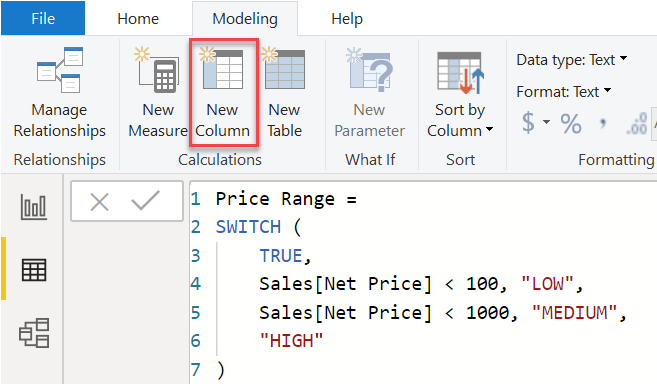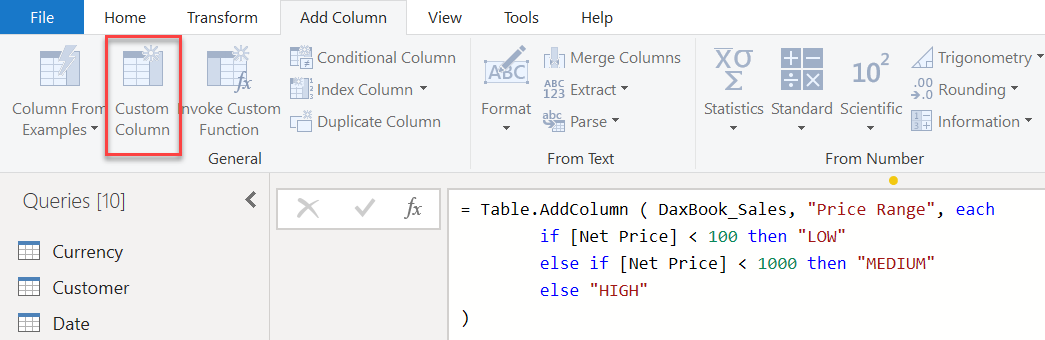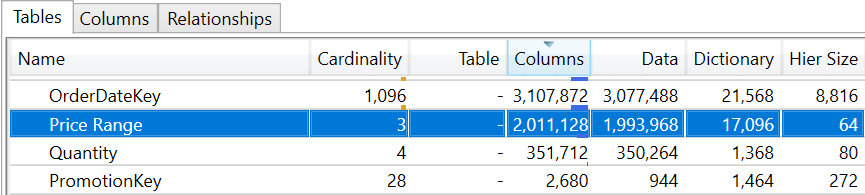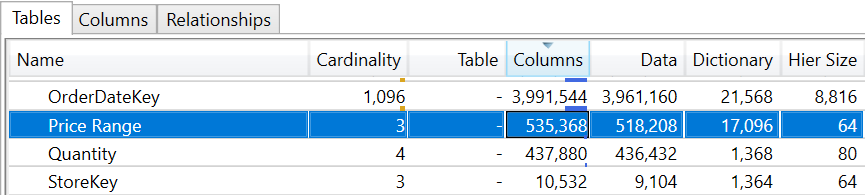## Introduction

Today we compare the power query computed columns with DAX calculated columns. When building a data model in Power BI, Analysis Services, or Power Pivot. You may need to extend a table with additional columns coming from a data source whose content is derived from existing data. Several features are available in the tools mentioned above for creating such additional columns. Choosing the right feature can sometimes be a challenge.

This article describes the most variations between calculated columns supported DAX expressions and computed columns generated by Power query. As you’ll see, the choice of a DAX calculated column ought to be restricted. Wherever the result’s obtained by accessing data in several rows of the same table or different tables, you should select a power query computed column, once the business logic to implement, depends on the values of other columns of the same table.

IMPORTANT: A DAX calculated column in an exceedingly DirectQuery model is evaluated at query time equally to what happens with Power query computed columns. Most of the Power BI consultants and Experts advice to applies only to models in which you import data into the memory.

## Introducing DAX Calculated Columns

A DAX calculated column computes the results of a DAX expression when the content of the model imported in memory. The DAX expression executed for every row of a table can use columns of the same table through the row context and can aggregate data using the filter context that is initially empty. The results of the DAX expression evaluate for each row are kept in memory as other columns of the table; this result utilized in other calculations and to filter and group data. The following screenshot shows a column calculated with Price Range in the Sales table, which depends only on the Sales [Net Value] column values.## Introducing Power Query Computed Columns

A computed Power Query column is an expression M which defines the transformations to be applied to a data source before importing a table into the data model. Depending on the expression and the source of the data, the M expression of a computed Power Query column can be converted into an analogous request to the data source – like the expression of a column in a SQL query; it can also be computed by the Power Query engine, changing the rows from the data source used to fill the data model table. The following screenshot shows a calculated Price Range column in the Sales table query, which depends on the Net Price column value.## Differences Between Power Query Computed Columns and DAX Calculated Columns

List of the most important differences between columns calculated with DAX and columns calculated with Power Query.

• Adding a column to the model
• A new DAX calculated column doesn’t need a full refresh of the table.
• A new Power Query computed column needs a full refresh of the table.
• Full refresh of the table
• The partition’s data have been loaded and compressed in memory when the engine processes DAX calculated columns.
• A Power Query compute column can be processed like every other column returning from the data source.
• Incremental refresh and partition refresh
• The engine processes the DAX calculated columns for all the rows of the table, even once only a couple of rows added to or refreshed in the table.
• The engine only computes values for the Power Query computed columns of the rows that processed in any partial refresh.
• Comparing compression
• A DAX calculated column doesn’t participate within the evaluation of the best sort order for the compression. As a result, the compression of the column might be far from ideal, especially for columns with a low number of unique values.
• A Power Query computed column compressed like all different columns of the table.
• Processing time
• The cost of processing a DAX calculated column corresponds to an ordered analysis of the DAX expression for every row included in a refresh operation. Within the same table, Multiple DAX calculated columns processed sequentially.
• The cost of processing a Power Query calculated column mostly depends on the data source. It depends on when the expression optimized using query folding. For example, if M expressions translated into equivalent SQL expressions. The performance and parallelism entirely depend on the SQL data source.
• Improving the compression of one column might negatively impact the compression of other columns in the data model.
• Query performance
• The column with higher compression is smaller in memory and frequently provides higher performance levels. This is necessary for the filter, group, and aggregation operations involving the column.
• The compression of a DAX calculated column may be lower than that of a Power Query computed column.
• An increase in one column compression could have a negative effect on the compression of other columns in the data model.

## Explaining Different Compression Results

A measured column with a small number of unique values does not get the advantage of changing the table sort order. In fact, that results in less effective compression. For example, in a Sales table with more than 12 million rows, we generated the Price Range column using the two techniques outlined above. VertiPaq Analyzer reveals that the calculated column size for the Price Range data in DAX is 1,993,968 bytes.By creating Price Range in Power Query as computed column, the data size is only 518,208 bytes.Because you delete approximately 75 percent of the Price Range column size, this doesn’t necessarily mean you saved the same number of bytes (1,475,760) from the entire database size. Certain columns can undergo a worse compression. For example, both the columns Quantity and OrderDateKey seen in the two preceding figures saw a significant increase in their size. Here the size of the model increased with the column computed, as you can see from the VertiPaq files available for download. Nonetheless, if you alter a single column in a layout, the result obtained is almost unpredictable. Calculated columns are generally a better choice, although the overall result may vary from case to case.

## When to Use Power Query Computed Columns

You should use Power Query computed columns. If the expression only depends on other columns of the same row of the table where you create the new column. The Price Range column used in this article’s example is the case.

If the source of data is a relational database and you add tables that can be effectively linked to the source of the data. It is a good idea to denormalize a table using Power Query functions–instead of importing several tables into the data model and then generating DAX measured columns with RELATED to retrieve related data from other tables.

When determining whether to use computed columns in Power Query, you should be careful, when rows need to be aggregated from other tables. The computed columns of the Power Query perform aggregation on the source of the data. This might take a long time to execute. If this occurs, consider a calculated column with DAX to avoid long processing times.

## When to Use DAX Calculated Columns

Whenever you can not implement equal measurements in a Power Query computed column, you should use calculated columns with DAX. The typical case is when the prototype aggregates data from other tables. Another case would be to denormalize tables that come from different sources of data.

## Conclusion

In this article, we have discussed the comparison among power query computed columns, and DAX calculated columns. If you have any queries or need support, get in touch with Power BI experts or comment in the comment section.

Happy learning!